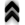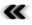## Appendix CRichardson Extrapolation

Digital computers cannot, in general, evaluate values with inﬁnite precision and thus need to rely on approximations. It is, of course, among the goals of any scheme resulting in such an approximate value to have as little an error as possible. Numerical schemes to reduce this error, such as the Richardson extrapolation presented here, are therefore of great interest.

The Richardson extrapolation scheme assumes that a function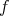can be expanded around a point of evaluation depending on a parameter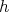in a form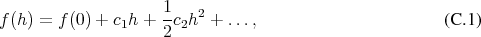such that the point of evaluation corresponds to the parameter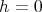. Expanding the function at two diﬀerent values of the parameter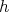and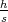gives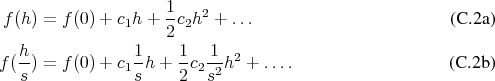Multiplying Equation  byand subtracting Equation  allows to eliminate the terms of order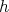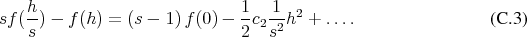Reordering this expression shows the approximation for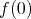explicitly as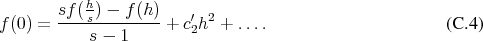An approximation which now is of order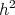instead of the original order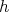. This procedure can be applied repeatedly to further increase the quality of the approximation.

Applications using digital computers usually utilize factorscorresponding to powers of. This allows the following settings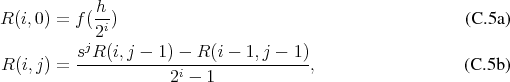which further illustrate the recursive nature of the procedure.